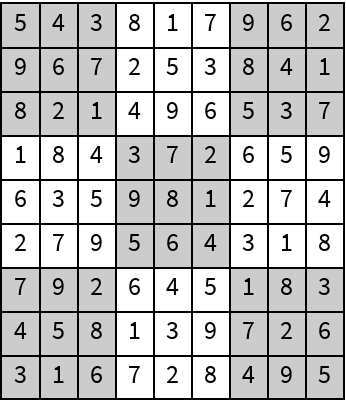#Function Repository Resource:

# GenerateSudokuPuzzle

Generate a sudoku puzzle

Contributed by: Paritosh Mokhasi
 ResourceFunction["GenerateSudokuPuzzle"][] generates a 9×9 sudoku puzzle with half of the elements exposed. ResourceFunction["GenerateSudokuPuzzle"][d] generates a d2×d2 sudoku puzzle with half of the elements exposed. ResourceFunction["GenerateSudokuPuzzle"][d,p] generates a d2×d2 sudoku puzzle with p percent of the elements exposed.

## Details and Options

The first argument specifies the size of the sudoku puzzle and must be a positive integer.
The second argument specifies the percentage of elements to be exposed. The second argument must be a number between 0 and 1.
The function generates a sudoku puzzle by starting with a full sudoku puzzle. From this, a randomly selected element from the puzzle is removed. The resulting puzzle is then solved with the additional constraint that the number removed cannot exist at that position. If the solver returns a result, it means that the solution is not unique and that element cannot leave the puzzle. If the solver comes back with a failed result, then that number at that position is unique and can be removed.
The function returns a two-element list. The first element contains the completed/reference sudoku puzzle and the second element is the actual sudoku puzzle for solving.

## Examples

### Basic Examples

Generate a standard sudoku puzzle:

 In:=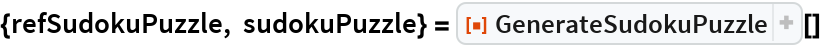Out=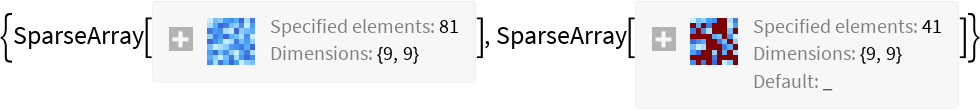In:=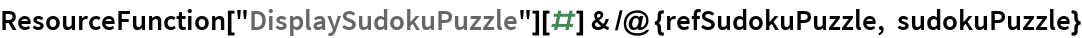Out=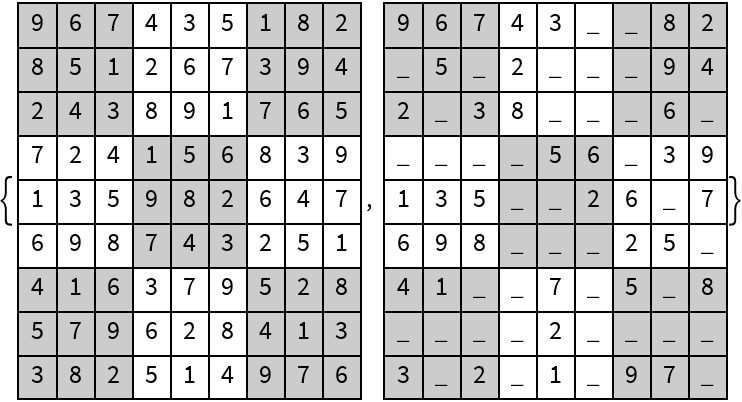Solve the resulting sudoku puzzle to confirm that the reference puzzle is obtained:

 In:=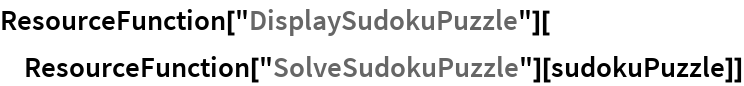Out=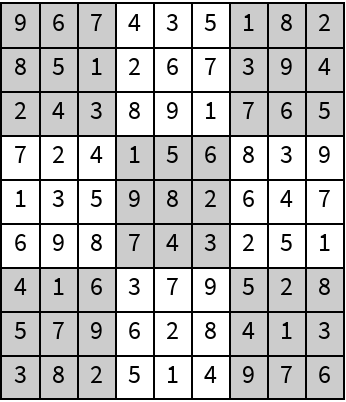Generate a 16×16 sudoku puzzle with 70% of the elements exposed:

 In:=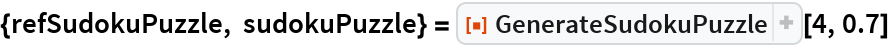Out=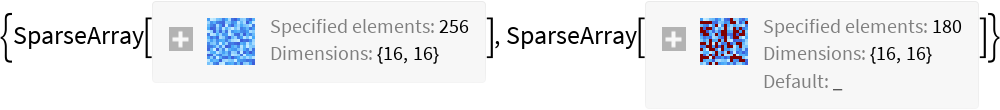In:=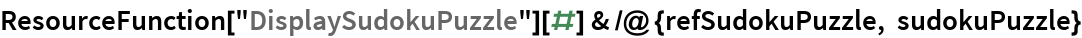Out=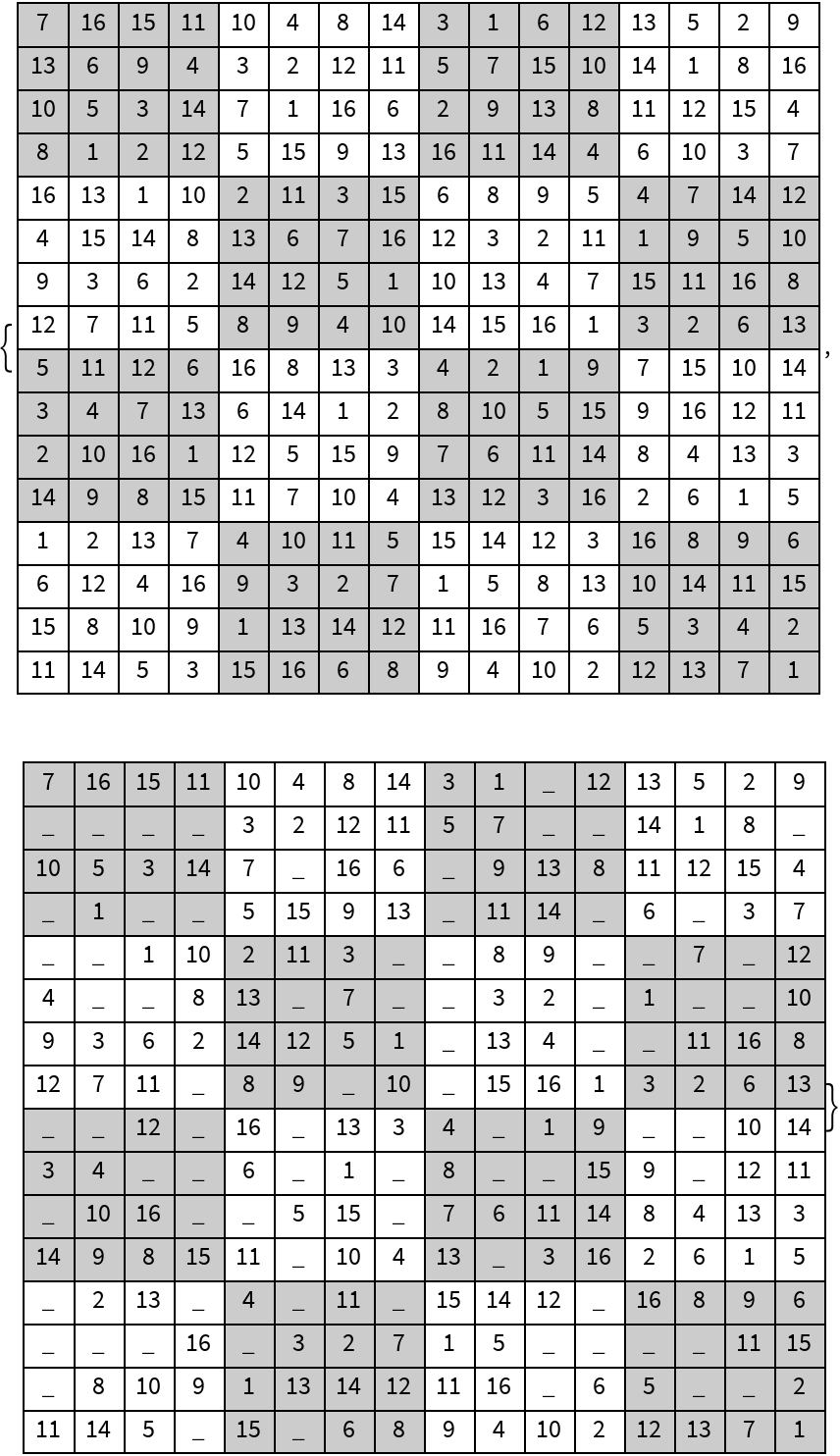### Possible Issues

If the specified exposed percentage is too low, then the function will return a puzzle with the smallest number of elements exposed such that the resulting sudoku puzzle is unique:

 In:=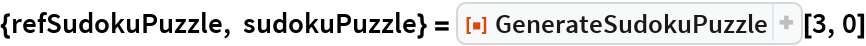Out=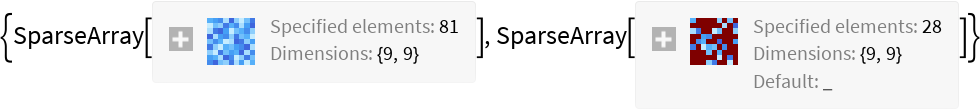In:=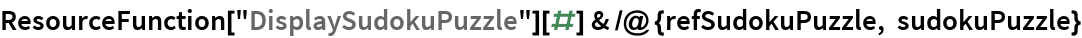Out=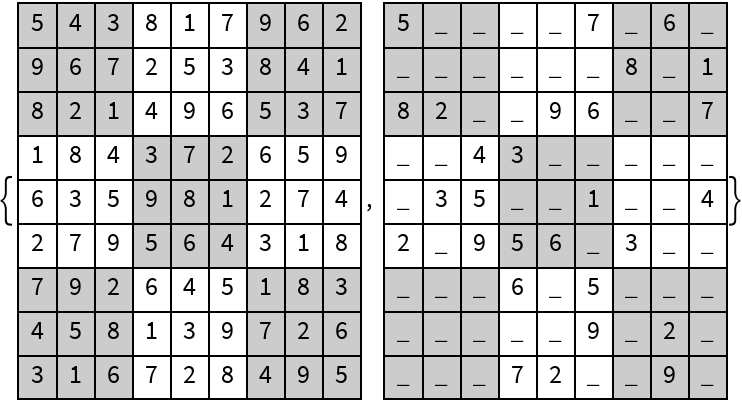Solve the resulting sudoku puzzle to confirm that the reference puzzle is obtained:

 In:=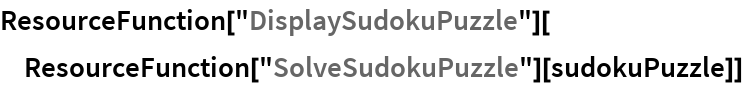Out=